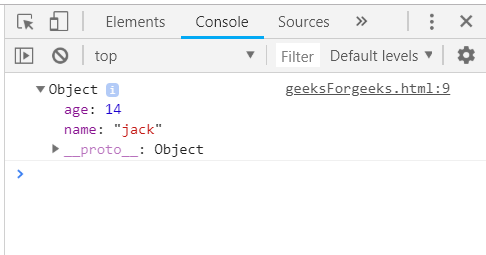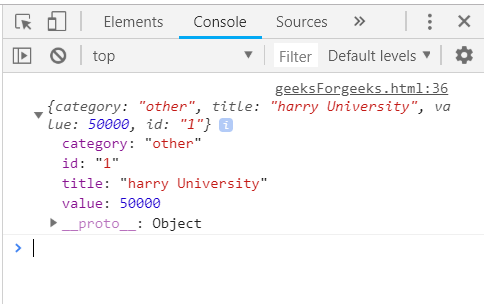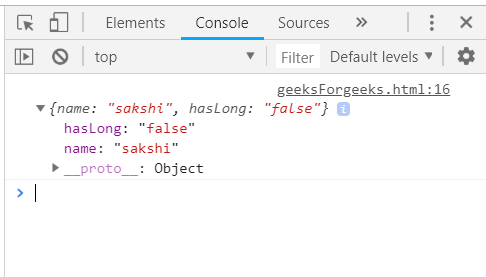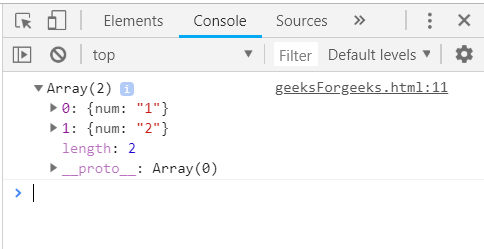Open In App
Related Articles

# Underscore.js _.first() Function

The Underscore.js  _.first() function is used to return the first element of the array, i.e. the number at the zeroth index. It returns the first n elements in the array of m size (n < m) by just passing the variable n in the array. It is a very easy-to-use function of the underscore.js library and is used widely when working with array elements.

Syntax:

`_.first(array, [n]) `

Parameters: This function accepts two parameters as mentioned above and described below:

• array: This parameter is used to hold the array of elements.
• variable: It tells the number of elements wanted.

Return values: This function returns the array of elements.

Passing an array to the _.first function(): The ._first() function will return the first element along with all its properties of the array passed. Like here, the elements have 2 properties, the name, and the age so, the final result will contain both of these properties of the first element as the variable n is not passed here.

Example:

## html

 `<``script` `type``=``"text/javascript"` `src``=``"https://cdnjs.cloudflare.com/ajax/libs/underscore.js/1.9.1/underscore.js"``>```` ` `<``script` `type``=``"text/javascript"``>``    ``console.log(_.first([{name: 'jack', age: 14},``                        ``{name: 'jill', age: 15},``                        ``{name: 'humpty', age: 16}]));```

Output:Passing a structure to _.first() function: The ._first() function will return the first element along with all its properties of the array passed as the variable n is not passed here. Like here, each element has four properties, the category, the title, the value, and the id. So, the final result will contain all of these properties of the first element.

Example:

## html

 `<``script` `type``=``"text/javascript"` `src``=``"https://cdnjs.cloudflare.com/ajax/libs/underscore.js/1.9.1/underscore-min.js"``>```` ` `<``script` `type``=``"text/javascript"``>``    ``var goal = [``    ``{``        ``"category" : "other",``        ``"title" : "harry University",``        ``"value" : 50000,``        ``"id":"1"``    ``},``    ``{``        ``"category" : "travelling",``        ``"title" : "tommy University",``        ``"value" : 50000,``        ``"id":"2"``    ``},``    ``{``        ``"category" : "education",``        ``"title" : "jerry University",``        ``"value" : 50000,``        ``"id":"3"``    ``},``    ``{``        ``"category" : "business",``        ``"title" : "Charlie University",``        ``"value" : 50000,``        ``"id":"4"``    ``}``            ``]``            ``console.log(_.first(goal));```

Output:Passing an array with one property as true/false to the _.first() function: This will work exactly the same as the above two examples. The false/true property will only be displayed in the first element. Here, this property will not be logically used.

Example:

## html

 `<``script` `type``=``"text/javascript"` `src``=``"https://cdnjs.cloudflare.com/ajax/libs/underscore.js/1.9.1/underscore-min.js"``>```` ` `<``script` `type``=``"text/javascript"``>``    ``var people = [``    ``{"name": "sakshi", "hasLong": "false"},``    ``{"name": "aishwarya", "hasLong": "true"},``    ``{"name": "akansha", "hasLong": "true"},``    ``{"name": "preeti", "hasLong": "true"}``            ``]``            ``console.log(_.first(people));```

Output:Passing an array with the variable n to the _.first() function: To have more than one element which is the first element, just pass the number and get a result. Remember that the elements will always come from the start of the array.

Example:

## html

 `<``script` `type``=``"text/javascript"` `src``=``"https://cdnjs.cloudflare.com/ajax/libs/underscore.js/1.9.1/underscore-min.js"``>```` ` `<``script` `type``=``"text/javascript"``>``    ``var users = [{"num":"1"}, {"num":"2"},``    ``{"num":"3"}, {"num":"4"}, {"num":"5"}];``            ``console.log(_.first(users, 2));```

Output: